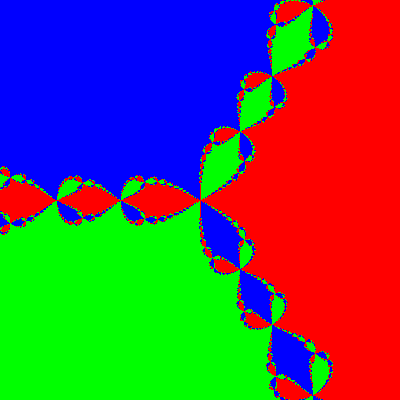# Basin Of AttractionAllPages RecentChanges Links to this page Edit this page Search Entry portal Advice For New UsersBasins of attraction of the cubic $x^3=1$
The basin of attraction of a particular solution to an equation, such as a cubic equation, under a solution method, such as Newton's Method, is the collection of starting points that end at that solution.

For the cubic equation in the complex plane the basins of attraction are fractals.

Here we have coloured each point according to the root that Newton's Method converges to. When close to a root, that's where you end up. However, when nearly equidistant from two roots the derivative is close to zero, so the tangent can take us a long way away. Then we are starting the process from an effectively random point.